Number Sequences 1

What is the 9th:
a) Odd number; 17
b) Square number; 81
c) Prime number. 23

Factors

Find all the factors of:

47

1, 47.

Multiples

Subtract the 4th from the 7th multiples of:

12

36

Polygons

What are the names of regular polygons with:
a) five sides;
b) six sides;
c) seven sides.

Pentagon, Hexagon and Heptagon (all regular)

Rounding

Round the following numbers to three significant figures:
a) 33.64; 33.6
b) 914610; 915000
c) 0.005895; 0.00590

Area of a Triangle

Find the area of a triangle that has a base of 4cm and a height of 8cm.

16cm2

Area of a Trapezium

Find the area of a trapezium that has a base of 13cm, a height of 12cm and a top (parallel to base) of 7cm. 120cm2

Evaluate:

$$\frac{3}{5} + \frac{6}{9}$$ $$= 1\frac{4}{15}$$

Fractions (Multiplying)

Evaluate:

$$\frac{2}{4} × \frac{5}{6}$$ $$= \frac{5}{12}$$

Fractions (Dividing)

Evaluate:

$$\frac{3}{5} ÷ \frac{8}{7}$$ $$= \frac{21}{40}$$

Circle (Vocabulary)

Name the red part.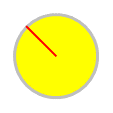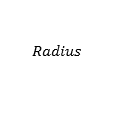Venn Diagrams

Describe the red region.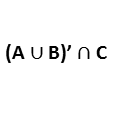Shape Formulas

What is the formula?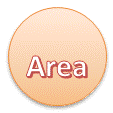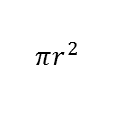What is it?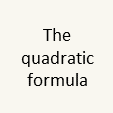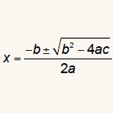Fraction to Percentage

Convert this fraction to a percentage.

$$\frac{3}{5}$$ $$= 60$$%

Circle Area

Find the area of a circle that has a radius of 1cm. Give your answer to three significant figures.

3.14cm2

Circle Circumference

Find the circumference of a circle that has a radius of 7cm. Give your answer to three significant figures.

44.0cm2

Calculate the value of:

3.6 + 7.4

= 11

Decimals (Subtracting)

Calculate the value of:

5.2 − 2.6

= 2.6

Decimals (Multiplying)

Calculate the value of:

2.2 × 4.7

= 10.34

Decimals (Dividing)

Calculate the value of:

93.5 ÷ 17

= 5.5

Indices (Simple)

What is the value of:

52

= 25

What is the value of:

3-3

= 0.037037037037037

Calculate the value of:

86 + 74

= 160

Basic Subtraction

Calculate the value of:

54 − 29

= 25

Basic Multiplication

Calculate the value of:

57 × 65

= 3705

Basic Division 2

Calculate the value of:

576 ÷ 12

= 48

Percentage (Of)

Find the value of:

5% of 140

= 7

Standard Form 1

Find the value of:

7.14 × 105

= 714000

Highest Common Factor

Find the highest common factor of forty five and fifteen.

= 15

Times Tables (2-5)

 5 × 2 = 10 6 × 4 = 24 3 × 3 = 9 9 × 3 = 27 7 × 2 = 14 4 × 5 = 20 8 × 5 = 40 2 × 4 = 8

Times Tables (2-12)

 4 × 12 = 48 9 × 12 = 108 5 × 6 = 30 6 × 5 = 30 7 × 8 = 56 3 × 6 = 18 8 × 6 = 48 2 × 8 = 16

Times Tables (2)

 5 × 2 = 10 7 × 2 = 14 3 × 2 = 6 6 × 2 = 12 9 × 2 = 18 8 × 2 = 16 4 × 2 = 8 2 × 2 = 4

Times Tables (3)

 4 × 3 = 12 9 × 3 = 27 7 × 3 = 21 6 × 3 = 18 3 × 3 = 9 8 × 3 = 24 5 × 3 = 15 2 × 3 = 6

Times Tables (4)

 3 × 4 = 12 5 × 4 = 20 8 × 4 = 32 6 × 4 = 24 9 × 4 = 36 4 × 4 = 16 7 × 4 = 28 2 × 4 = 8

Times Tables (5)

 6 × 5 = 30 3 × 5 = 15 8 × 5 = 40 5 × 5 = 25 4 × 5 = 20 9 × 5 = 45 7 × 5 = 35 2 × 5 = 10

Times Tables (6)

 7 × 6 = 42 4 × 6 = 24 8 × 6 = 48 3 × 6 = 18 6 × 6 = 36 9 × 6 = 54 5 × 6 = 30 2 × 6 = 12

Times Tables (7)

 8 × 7 = 56 6 × 7 = 42 4 × 7 = 28 9 × 7 = 63 7 × 7 = 49 3 × 7 = 21 5 × 7 = 35 2 × 7 = 14

Times Tables (8)

 8 × 8 = 64 3 × 8 = 24 4 × 8 = 32 5 × 8 = 40 6 × 8 = 48 7 × 8 = 56 9 × 8 = 72 2 × 8 = 16

Times Tables (9)

 6 × 9 = 54 7 × 9 = 63 8 × 9 = 72 3 × 9 = 27 4 × 9 = 36 5 × 9 = 45 9 × 9 = 81 2 × 9 = 18

Times Tables (12)

 6 × 12 = 72 5 × 12 = 60 7 × 12 = 84 9 × 12 = 108 8 × 12 = 96 4 × 12 = 48 3 × 12 = 36 2 × 12 = 24

Fractions (Equivalent)

Write this fraction in its simplest form:

$$\frac{8}{32}$$ $$= \frac{1}{4}$$

Fractions (Mixed)

Evaluate:

$$2\frac{2}{3} − \frac{5}{6}$$ $$= 1\frac{5}{6}$$

Pythagoras

Find BC if AB = 5.4m and AC = 6.8m. 8.68m

Trigonometry (Angle)

Find angle BCA if AC = 3.8m and BC = 5.8m. 49.1o

Trigonometry (Side)

Find BC if angle BCA = 62o and AC = 4.5m. 9.59m

2

2

2

Fraction to Decimal

Convert this fraction to a decimal.

$$\frac{1}{2}$$ $$= 0.5$$

Decimal to Fraction

Convert this decimal to a fraction.

$$0.77$$ = $$\frac{77}{100}$$

Percentage (Increase)

Increase £140 by 35%

£189

Lowest Common Multiple

What is the lowest common multiple of eight and fourteen.

= 56

Sequence (Arithmetic)

5,14,23,32,41...

Find the:
a) next term; 50
b) nth term; 9n - 4
c) term number 35; 311

Sequence (Geometric)

5,20,80,320,1280...

Find the:
a) next term; 5120
b) nth term; 5 × 4n-1
c) term number 8; 81920

Interest (Simple)

If £160 is invested for 5 years with a simple interest rate of 6%, find the amount of interest earned. £48.00

Interest (Compound)

If £180 is invested with an interest rate of 4% compounded annually, find the value of the investment after 5 years. £219.00

Currency Exchange

If £1 is worth $1.45, convert: a) £200 to dollars;$290.00

b) \$220 to pounds; £137.93

Coordinates (Midpoint)

What are the coordinates of the midpoint of the line joining:

$$(0,3) \text{ and } (10,13)$$

(5,8)

What is the gradient of the line joining:

$$(7,-8) \text{ and } (11,-2)$$

$$\frac{3}{2}$$

Coordinates (Square)

Here are the coordinates of 3 vertices of a square, what are the coordinates of the 4th?

$$(4,5),(10,8),(1,11)$$

(7,14)

Negative Numbers

a) 12 − 23 = -11
b) 12 × (-10) = -120
c) (9−17)(7−13) = 48
d) 120 ÷ (-10) = -12
e) (-5)2 = 25

Substitution

If p = 5, q = 29 and
r = -8 evaluate:

a) 2q − p = 53
b) pq + r = 137
c) p2 − 5q - r = -112

Equations (Type 1)

Solve:

$$3x = 15$$

$$x = 5$$

Equations (Type 2)

Solve:

$$4x +9= 29$$

$$x = 5$$

Equations (Type 3)

Solve:

$$4x +3= 2x + 9$$

$$x = 3$$

Equations (Type 4)

Solve:

$$4(2x -4)-9= 15$$

$$x = 5$$

Equations (Type 5)

Solve:

$$5(2x + 4)= 4(5x + 5)$$

$$x = 0$$

Equations (Simultaneous 1)

Solve:

$$2x-4y = -6$$
$$3x-4y = 1$$

$$x = 7, y = 5$$

Equations (Simultaneous 2)

Solve:

$$5x+5y = 30$$
$$5x+20y = 60$$

$$x = 4, y = 2$$

Equations (Simultaneous 3)

Solve:

$$6x-2y = -10$$
$$7x-7y = 14$$

$$x = -3.5, y = -5.5$$

Sets (Union)

Find the union of:

{1,3,5,7,9} and
{2,6,12}

{1,2,3,5,6,7,9,12}

Sets (Intersection)

Find the intersection of:

{1,2,3,4,5} and
{6,7,8,9,10}

Bearings

A plane flies from point A to point B on a bearing of 346o. What bearing would it return on from B to A? 166o

Probability

A number is picked at random from the set

{2,4,6,8,10}

what is the probability it is even? 1

Evaluate:

28 ÷ 7 × 42 ÷ 6

28

Simplify

Simplify the following by collecting like terms:

$$2y+2y^2-5y+y^2$$

$$3y^2-3y$$

Ratio

Divide 105 in the ratio

8:7

56 and 49

Graph (Linear)

Draw a rough sketch of the graph of:

$$y=x-2$$

y intercept -2

Prime Factors

Express the following number as the product of prime numbers:

45

3 x 3 x 5

Percentage (Reverse)

In a sale an item costs £48 after a 20% reduction. What was the original price?

£60

Averages

Find the mean, mode, median and range of the following:

1,2,3,4,5

Mean = 3, no mode,
median = 3 and range = 4

Time (Analogue)

What time is this?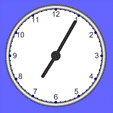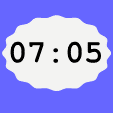Time (Digital)

Sketch a clock face: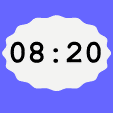Decimals (Recurring)

Write the following recurring decimal as a fraction in its lowest terms.

0.929292... $$\frac{92}{99}$$

Percentage (Decrease)

Decrease £80 by 40%

£48

Brackets (Linear)

Expand:

$$2(2x-8)$$

$$4x-16$$

Expand:

$$(x+1)(4x-2)$$

$$4x^2+2x-2$$

Factorise (Linear)

Factorise:

$$27x-24$$

$$3(9x-8)$$

Factorise:

$$x^2-2x-8$$

$$(x+2)(x-4)$$

Factorise:

$$5x^2+7x-6$$

$$(x+2)(5x-3)$$

Circle Theorems

Which theorem?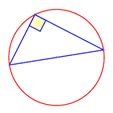Standard Form 2

Find the value of:

7.74 × 10-5

= 0.0000774

Standard Form 3

Write in standard form:

8840

= 8.84 × 103

Standard Form 4

Write in standard form:

0.000743

= 7.43 × 10-4

Find the nth term:

$$0, 10, 24, 42, 64,$$

$$2n^2+4n-6$$

Standard Form 5

Multiply 9 × 103
by 9 × 104 and give the answer in standard form.

= 8.1 × 108

Solve:

$$x^2+x-12= 0$$

$$x = 3$$ and $$-4$$

Solve this equation giving the solutions to 3 significant figures:

$$4x^2-5x-4 = 0$$

$$x = 1.80$$ and $$-0.554$$

Polygon Angles

What is the size of each exterior angle of a regular hexagon?

60°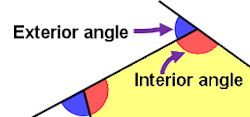Change The Subject

Make $$b$$ the subject of the formula
$$c=3b-5$$

$$b=\frac{c+5}{3}$$

Basic Division 1

Calculate the value of:

4068 ÷ 6

= 678

Number Sequences 2

What is the 8th:
a) Cube number; 512
b) Triangular number; 36
c) Fibonacci number. 21

A Mathematics Lesson Starter Of The Day

Share
• Jan, South Canterbury
•
• Thank you for sharing such a great resource. I was about to try and get together a bank of starters but time is always required elsewhere, so thank you.
• Barbara Schindler, Newton Rigg College
•
• I use Refreshing Revision a lot but today all the brackets and fractions keep coming up 'jumbled' . There are curly brackets, part words / 's in odd places and it is impossible to make out the question. It is doing this on 2 computers I have tried. Can you help? thank you.

[Transum: Sorry to hear about this problem Barbara. I have tested it from here and it seems to be working OK. Please take a look at the MathJax FAQ. Many apologies for the inconvenience.]
• Lesley, UK
•
• Answers for the starter would be great so students can get immediate feedback and become independent learners.

[Thanks for your comments Lesley. The answers are only available to signed-in teachers and parents I'm afraid. I you are a subscriber and are projecting this Starter for the whole class to see you can scroll down the page and show the same questions with the answers included in red.]
• Mrs B, Stockport
•
• Refreshing Revision really useful resource, that I have actually used for Ks2 revision as some topics are appropriate. I'd love to see either ks2 version, with purely ks2 SATs level topics or adding them to the current version.

[Transum: Thanks so much for your feedback Mrs B. If you could send me a list of your top ten ideas for topic you would like to see added I will work on it]
• Mark Adams, St Peters RC School Solihull
•
• It would be great if these questions came with answers as well.

[Transum: The answers to Transum activities are available to those signed in to their Transum subscription account. You can sign up for an account here.]

How did you use this starter? Can you suggest how teachers could present or develop this resource? Do you have any comments? It is always useful to receive feedback and helps make this free resource even more useful for Maths teachers anywhere in the world.

If you don't have the time to provide feedback we'd really appreciate it if you could give this page a score! We are constantly improving and adding to these starters so it would be really helpful to know which ones are most useful. Simply click on a button below:

Excellent, I would like to see more like this
Good, achieved the results I required
Satisfactory
Didn't really capture the interest of the students
Not for me! I wouldn't use this type of activity.

This starter has scored a mean of 3.2 out of 5 based on 505 votes.

Previous Day | This starter is for 9 April | Next Day

Concept Selection

Tick (or untick) the boxes above to select the concepts you want to be included in this Starter. The display at the top of this page will change instantly to show your choices. You can also drag the panels above so that the questions are ordered to meet your needs.

This Starter is called Refreshing Revision because every time you refresh the page you get different revision questions.

Regularly use this Starter to keep that important learning from being forgotten. Here is the web address (URL) for the version of this page with your currently selected concepts:

Copy and paste the URL above into your lesson plan or scheme of work.

For more ideas on revision there are plenty of tips, suggestions and links on the Mathematics Revision page.

Your access to the majority of the Transum resources continues to be free but you can help support the continued growth of the website by doing your Amazon shopping using the links on this page. Below is an Amazon search box and some items chosen and recommended by Transum Mathematics to get you started.The analytics show that more and more people are accessing Transum Mathematics via an iPad as it is so portable and responsive. The iPad has so many other uses in addition to solving Transum's puzzles and challenges and it would make an excellent gift for anyone.

The redesigned Retina display is as stunning to look at as it is to touch. It all comes with iOS, the world's most advanced mobile operating system. iPad Pro. Everything you want modern computing to be. more...

I had been tutoring the wonderful Betsy for five years. When the day came for our last ever session together before the end of her Year 13, I received this beautiful book as a gift of appreciation.

This a very readable book by Ben Orlin. I'm really enjoying the humour in the writing and the drawings are great.

Ben Orlin answers maths' three big questions: Why do I need to learn this? When am I ever going to use it? Why is it so hard? The answers come in various forms-cartoons, drawings, jokes, and the stories and insights of an empathetic teacher who believes that mathematics should belong to everyone.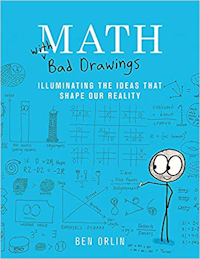Teacher, do your students have access to computers?Do they have iPads or Laptops in Lessons? Whether your students each have a TabletPC, a Surface or a Mac, this activity lends itself to eLearning (Engaged Learning).Transum.org/go/?Start=April9

Here is the URL which will take them to a related student activity.

Transum.org/go/?to=topictestTeacher:
Scroll down the
page to see how
this Starter can be customised so that it
is just right for